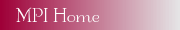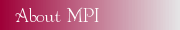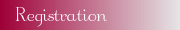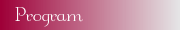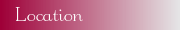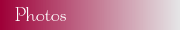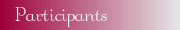# Do the Barker Codes End?

### Greg Coxson, Technology Service Corporation (TSC)

One of the older unsolved problems in radar and communications is that of determining whether there are any binary Barker codes longer than 13. The case of odd N was closed in a 1961 paper by R. Turyn in the Proceedings of the AMS. That paper detailed the structure of odd-length Barker codes and proved there were none longer than 13. However, the even case was left open, and remains open.

The Barker property is related to the autocorrelation function for binary codes. Consider a sequence x with N elements xi which can take the value +1 or -1. The most compact definition of the autocorrelation is x*rev(x), where * is a periodic convolution and rev( ) performs a reversal of x (the fliplr operator in MatLab). The autocorrelation has length 2N - 1, and the peak, ACF(N), is always N. The other elements are termed sidelobes, and the Barker codes are those for which max{k~=N}|ACF(k)| <= 1. This is the lowest possible peak sidelobe level for binary +/-1 codes.

There are a number of ways to approach this problem. One way is to generalize to polyphase codes in which elements can be mth roots of unity for some m > 2. In this case, the autocorrelation is defined as x*rev(conj(x)) where conj( ) means complex conjugation; the definition of peak sidelobe level becomes the greatest sidelobe magnitude. Now there are two code parameters to work with the length N, and this m. Search efforts suggest that as N grows, it becomes harder to find Barker codes regardless of m. However, Ein-Dor, et al.  use a statistical approach to suggest that there is a Barker sequence of any length as long as N = m. If this conjecture is true, then of course Barkers can be found at any length. The longest Barker found by optimization-oriented computer searches is for N = 77, by Carroll Nunn of TSC. Experience from search efforts suggests that as lengths grow, more decimal places are needed in order to represent these Barkers. This seems to go along with the Ein-Dor conjecture, that is, as N grows, the alphabet needs to grow.

There are numerous ways to choose the alphabet. One of many alternatives to m^th roots of unity is to control the number of decimal places in the representation of the code elements. Suppose you allow no decimal places in precision; then you have the set {1,-1,i, -i}. What happens if you allow one more decimal place? Then you get eight more points due to exactly one nondegenerate Pythagorean triple (x/10,y/10,1), x, y positive integers between 0 and 10. Add another decimal place, and you get 8 more points corresponding to a new Pythagorean triple (x/100,y/100,1) for x,y positive integers between 0 and 100. This pattern continues with each added decimal place. This gives some unexpected control over the problem. Even so, settling the Barker conjecture on such sets involves determining whether a collection of related sums of pairwise products of members of this set can all lie in the closed unit disk. It is daunting, but perhaps only due to lack of inspired mathematical approaches.

References

 Ein-Dor, et al., Low autocorrelated multiphase sequences, Physical Review E , 65 (2002).

Last Changed: Thursday, 15-May-2008 21:39:29 UTC
cims@wpi.edu